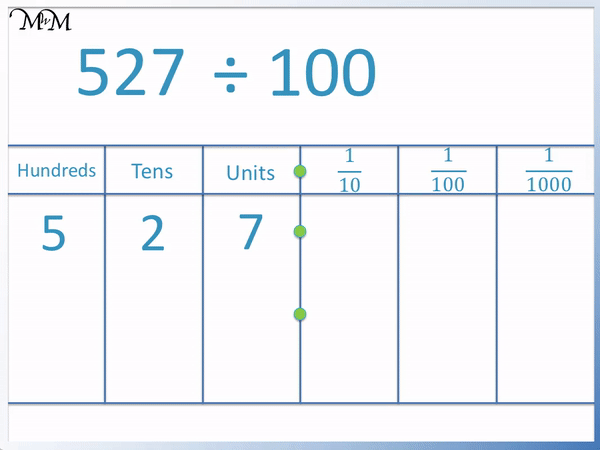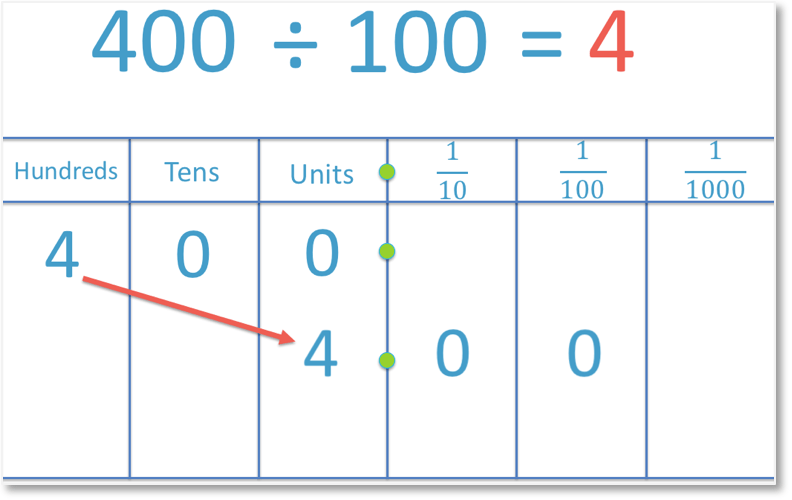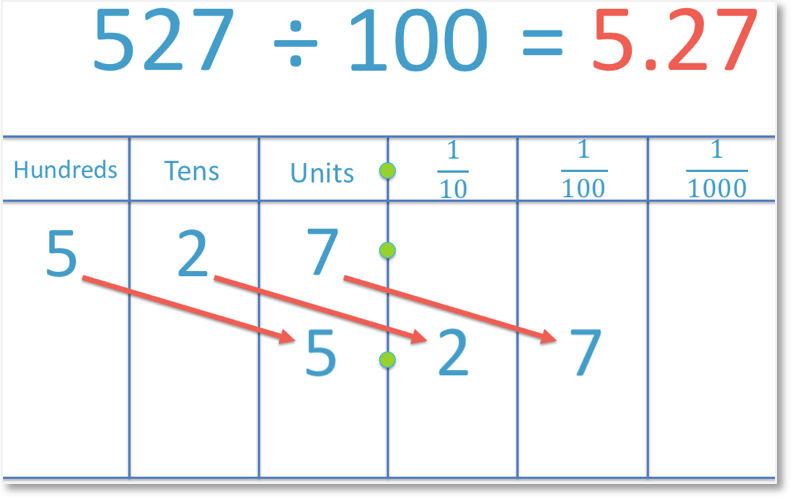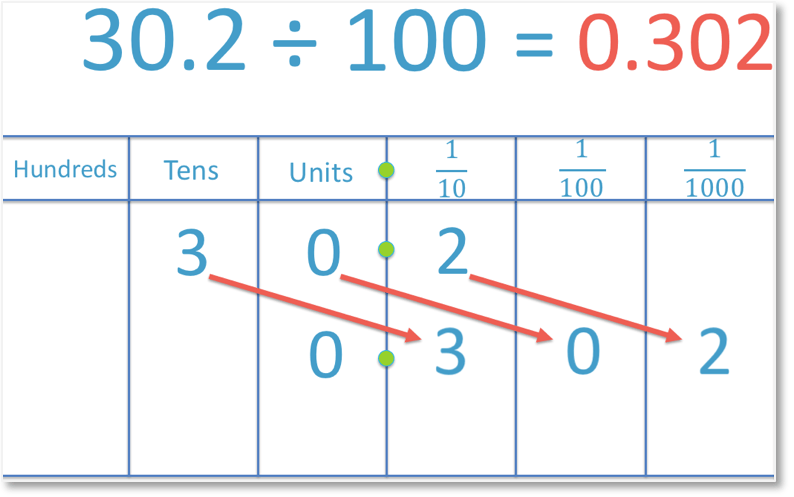# Dividing by 100

Dividing by 100• In this example we are dividing 400 by 100.
• To divide a number by 100, move all digits two place value columns to the right.
• The ‘4’ moves from the hundreds column to the units (ones) column.
• The ‘0’ in the tens column moves to the tenths column.
• The ‘0’ in the units column moves to the hundredths column.
• 400 ÷ 100 = 4.00
• If the only digits after the decimal point are zeros, then they should not be written.
• Therefore we can write: 400 ÷ 100 = 4.
• Dividing by 100 has the same effect of removing two ‘0’ digits only if the original number ended with two ‘0’ digits in the tens and units columns respectively.
To divide by 100, move each digit two place value columns to the right.

If the number ends in two ‘0’ digits in the tens and units columns, dividing by 100 has the same effect as removing these digits.• To divide by 100, move all digits two place value columns to the right.
• In this example, the digit ‘5’ moves from the hundreds column into the units column.
• The digit ‘2’ moves from the tens column into the tenths column.
• The digit ‘7’ moves from the units column into the hundredths column.
• 527 ÷ 100 = 5.27
• The digits ‘5’, 2′ and 7′ remain in the same order in the answer.Supporting Lessons#### Dividing by 100: Interactive Questions

Divide a Decimal by 100: Interactive Questions

# How to Divide a Number by 100

To divide a number by 100, move each digit in that number two place value columns to the right.

If the number ends with two zero digits in the tens and units column, then dividing by 100 has the same effect as removing these two zero digits.

This trick only works for whole numbers that are in the 100 times table (ending in two zeros).In the example above, we have 400 ÷ 100 = 4.

To divide a number by 100, move all of its digits two place value columns to the right.

The digit of ‘4’ moves from the hundreds column to the units column.

The digit of ‘0’ in the tens column moves to the tenths column, immediately after the decimal point.

The digit of ‘0’ in the units column moves to the hundredths column.

400 ÷ 100 is 4.00.

If a number only has zeros after its decimal point, then it is a whole number and there is no need to write these zeros.

It is better to write: 400 ÷ 100 = 4.

It can bee seen that we have simply “removed the two zeros”.

This trick only works for whole numbers that actually have two zeros in the tens an units columns to remove.

It is important to understand how dividing any number by 100 works because not all numbers that we divide by 100 will end in two zeros.

For example:To divide 527 by 100, we move each digit in 527 two places to the right.

We start by moving the ‘5’ from the hundreds column two places along into the units column.

The ‘2’ and the ‘7’ will then follow the ‘5’.

The 2 moves from the tens column into the tenths column and the 7 moves from the units column into the hundredths column.

Each digit has moved two places to the right and therefore 527 ÷ 100 = 5.27.

If the number’s largest digit is in the hundreds column, then this digit will move to the units column when it is divided by 100.

The remaining digits to the right will then appear in the same order to the right of the decimal point.

So in this example, the ‘5’ is the digit to the left of the decimal point and then the ‘2’ and the ‘7’ will be in the same order, immediately after the decimal point.

In the following example we are given a number that is less than 100 and has only two digits. We still follow the same rules for dividing by 100 and move each digit two places to the right.We begin by moving the ‘1’ two places to the right, from the tens column into the tenths column. The ‘9’ will then follow. We move it from the units column into the hundredths column.

Whenever there are no digits remaining to the left of the decimal point, a single ‘0’ digit is written in the units column.

Therefore, 19 ÷ 100 = 0.19.

We may be asked to divide a single-digit number by 100.

For example:Although 6 has only one digit, we still follow the same rule for dividing by 100. We move the 6 two places to the right. It moves from the units column into the tenths column.

Because there is no longer a digit in the units column and there is a space in the tenths column, we write a zero in both columns to show that their values are zero.

Therefore 6 ÷ 100 = 0.06.

We need to be careful when dealing with numbers where there is a zero between two other non-zero digits.

For example:To divide by 100, we must make sure that we move every digit in 30.2 two places to the right, including the zero. We move the 3 from the tens column into the tenths column, we move the zero from the units column into the hundredths column and we move the 2 from the tenths column into the thousandths column.

The digits remain in the same order. And so, 30.2 ÷ 100 = 0.302.Now try our lesson on Dividing by 1000 where we learn how to divide a number by 1000, with examples and further practice.